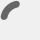### STM32F4 Pin Initialize without CMSIS

Category : Code

On York I/O board, there are 8 LEDs are connected PORT D.8 to D15. So, how can we initialize and drive those 8 leds or those pins via Keil IDE ?

I strongly advised to use CMSIS library for your further implementations. If you haven’t know what is CMSIS, please have a look at my CMSIS and TIMER on the ARM Cortex 4 Microcontroller tutorial. However, for some reasons if you are unable to use it, you can have a look below examples related with GPIO initialization.

This example is driving only one LED which is connected to PD.8

```</pre>
#include <stm32f4xx.h>

void LEDs_Init(void)
{
RCC->AHB1ENR |= RCC_AHB1ENR_GPIODEN; /*Enable the clock to GPIOD*/
GPIOD->MODER &= ~((3UL << 2*8)); /* Reset PD.8 pin's mode register*/
GPIOD->MODER |= ((1UL << 2*8) ); /* Assign "01" to PD.8 pin's mode register to initialize it as general purpose output */
GPIOD->OTYPER &= ~((1UL << 8) ); /* PD.8 output Push-Pull */
GPIOD->PUPDR &= ~((3UL << 2*8) ); /* PD.8 is Pull up */
GPIOD->PUPDR |= ((1UL << 2*8) );
}
void main(void)
{
LEDs_Init();
while(1)
{
//Do here whatever you want to do
GPIOD->ODR ^= (1UL << 8); //Toggling PD8 LED
}
}

```

Furthermore, if you want to drive all 8 LEDs which are connected to PD.8 to PD.15, you can modify previous code as below :

```</pre>
#include <stm32f4xx.h>

void LEDs_Init(void)
{
unsigned int ledNum = 8;
RCC->AHB1ENR |= RCC_AHB1ENR_GPIODEN; /*Enable the clock to GPIOD*/
for(ledNum = 8 ; ledNum < 16; ledNum++)
{
GPIOD->MODER &= ~((3UL << 2*ledNum)); /* Reset PD.8 pin's mode register*/
GPIOD->MODER |= ((1UL << 2*ledNum)); /* Assign "01" to PD.8 pin's mode register to initialize it as general purpose output */
GPIOD->OTYPER &= ~((1UL << ledNum)); /* PD.8 output Push-Pull */
GPIOD->PUPDR &= ~((3UL << 2*ledNum) ); /* PD.8 is Pull up */
GPIOD->PUPDR |= ((1UL << 2*ledNum)); /* Pull up and Pull down register setted to Pull-up*/
}
}

void main(void)
{
unsigned long temp = 0xFF;
LEDs_Init();
while(1)
{
//Do here whatever you want to do
GPIOD->ODR ^= (temp << 8); //Toggling 8 LEDs from PD8 to PD15
}
}
<pre>
```

Instead of for loop, you can do initialize multiple pins by more efficient way as well :

```</pre>
#include <stm32f4xx.h>

void LEDs_Init(void)
{

//Following dots should be filled as the following sequence. So do not complain about it is not compiled before filling this part.
RCC->AHB1ENR |= RCC_AHB1ENR_GPIODEN; /*Enable the clock to GPIOD*/
GPIOD->MODER &= ~((3UL << 2*8) | (3UL << 2*9) | ...| (3UL << 2*15) ); /* Reset PD.8..PD15 pins' mode register*/
GPIOD->MODER |= ((1UL << 2*8) | (3UL << 2*9) | ... |(3UL << 2*15) ); /* Assign "01" to PD.8..PD15 pins' mode register to initialize it as general purpose output */
GPIOD->OTYPER &= ~((1UL << 8) | (3UL << 2*9) | ... |(3UL << 2*15) ); /* PD.8..PD15 pins' output Push-Pull */
GPIOD->PUPDR &= ~((3UL << 2*8) | (3UL << 2*9) | ...| (3UL << 2*15) ); /* PD.8..PD15 pins' are Pull up */
GPIOD->PUPDR |= ((1UL << 2*8) | (3UL << 2*9) | ... |(3UL << 2*15) ); /* Pull up and Pull down register setted to Pull-up*/
}

void main(void)
{
unsigned long temp = 0xFF;
LEDs_Init();
while(1)
{
//Do here whatever you want to do
GPIOD->ODR ^= (temp << 8); //Toggling 8 LEDs from PD8 to PD15
}
}
<pre>```

Those pieces of code are not tested on a STM32F4 board so I hope it will not be any problem. If you have, please send a comment by below box.error: Content is protected !!